Tamilnadu State Board New Syllabus Samacheer Kalvi 8th Maths Guide Pdf Chapter 6 Statistics Ex 6.2 Text Book Back Questions and Answers, Notes.

## Tamilnadu Samacheer Kalvi 8th Maths Solutions Chapter 6 Statistics Ex 6.2

Question 1.
Which of the following data can be represented in a histogram?
(i) The number of mountain climbers in the age group 20 to 60 in TamilNadu.
Yes

(ii) Production of cycles in different years.
No(iii) The number of students in each class of a school.
No

(iv) The number votes polled from 7 am to 6 pm in an election.
Yes

(v) The wickets fallen from 1 over to 50th over in a one day cricket match.
YesQuestion 2.
Fill in the blanks:
(i) The total area of the histogram is _________ to the total frequency of the given data.
proportional

(ii) A graph that displays data that changes continuously over the periods of time is _________ .
Histogram

(iii) Histogram is a graphical representation of_________ data.
groupedQuestion 3.
In a village, there are 570 people who have cell phones. An NGO survey their cell phone usage. Based on this survey a histogram is drawn. Answer the following questions.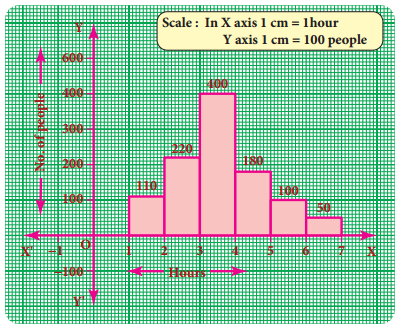(i) How many people use the cell phone for less than 3 hours?
330 people (110 + 220)

(ii) How many of them use the cell phone for more than 5 hours?
150 of them (100 + 50)

(iii) Are people using cell phone for less than 1 hour?
NoQuestion 4.
Draw a histogram for the following data.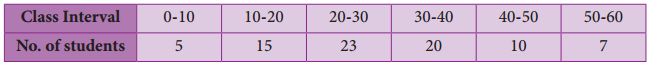The given (tata IS continuous frequency distribution taking class intervals on X axis and No. of students on Y-axis, the histogram is given below.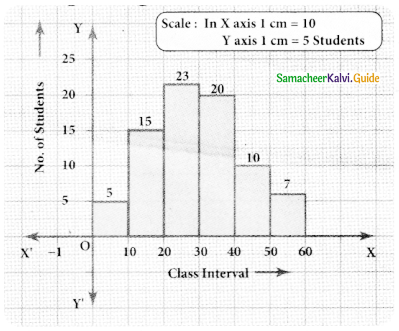Question 5.
Construct a histogram from the following distribution of total marks of 40 students in a class.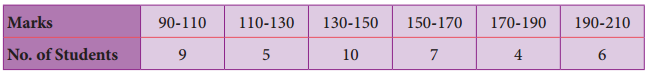The given distribution is continuous taking marks on X axis and No. of students on Y-axis the histogram is constructed.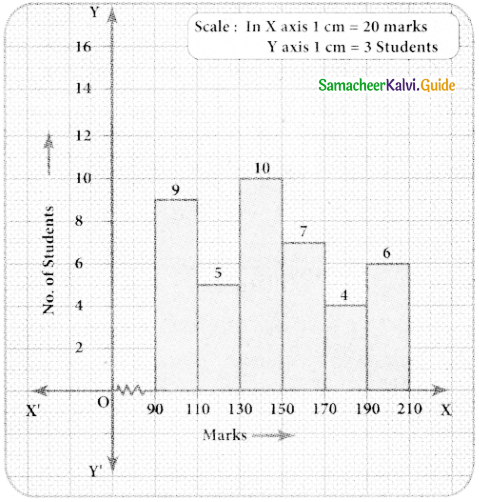Question 6.
The distribution of heights (in cm ) of loo people is given below. Construct a histogram and the frequency polygon imposed on it.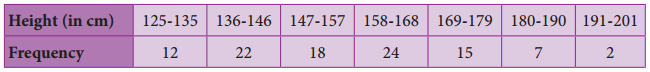The given distribution is discontinuous.
Converting into continuous distribution we have
Lower boundary = lower limit – $$\frac { 1 }{ 2 }$$ (gap between adjacent class interval)
= 125 – $$\frac { 1 }{ 2 }$$ (1) = 124.5
Upper boundary = Upper limit + $$\frac { 1 }{ 2 }$$ (gap between the adjacent class interval)
= 135 + $$\frac { 1 }{ 2 }$$ = 135.5
∴ The new frequency table is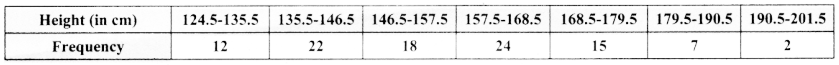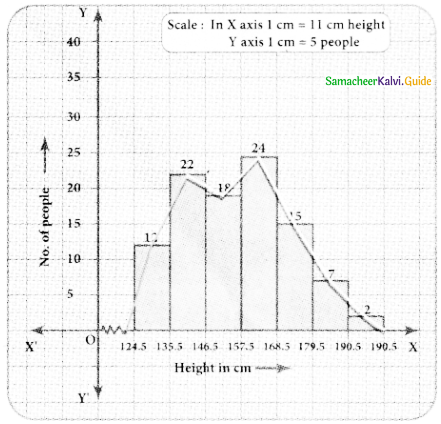Question 7.
In a study of dental problem, the following data were obtained.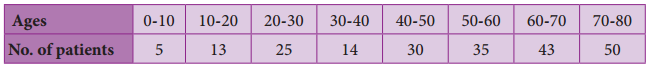Represent the above data by a frequency polygon.
Finding the midpoints of the class interval we get.

 Ages Mid point (x) No. of patients 0 – 10 5 5 10 – 20 15 13 20 – 30 25 25 30 – 40 35 14 40 – 50 45 30 50 – 60 55 35 60 – 70 65 43 70 – 80 75 50

The points tobe plotted are A(5,5), B(15, 13), C(25, 25), D(35, 14), E(45, 30), G(65, 43.), H(75, 50) to obtain the frequency polygon ZABCDEFGHI.
Where I imagined class between 80 and 90.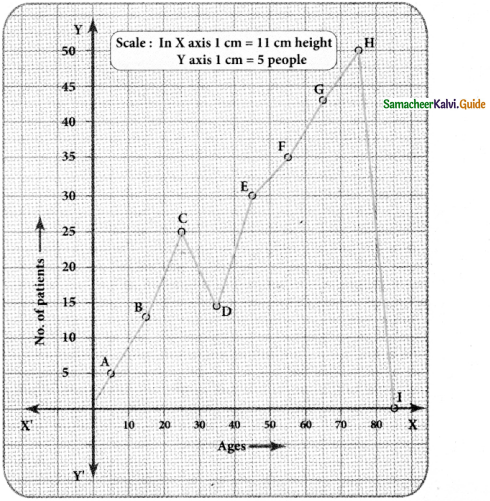Question 8.
The marks obtained by 50 students in Mathematics are given below (j) Make a frequency distribution table taking a class size of 10 marks (ii) Draw a histogram and a frequency polygon.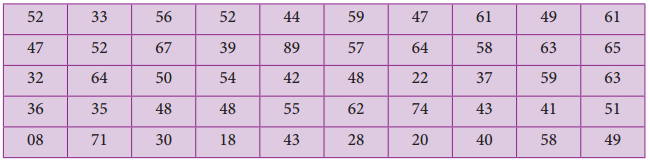Maximum marks obtained = 89
Minimum marks obtained = 08
Range = Maximum marks – Minimim marks
= 89 – 08
= 81
Taking the class size = 10, then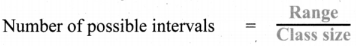= $$\frac{81}{10}$$ = 8.1 = 9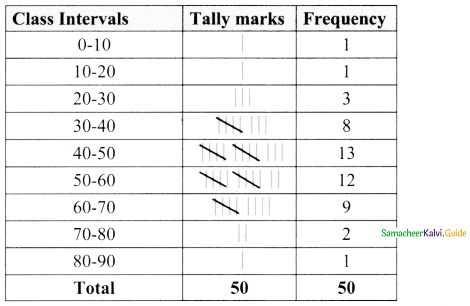Now we have the continuous frequency table.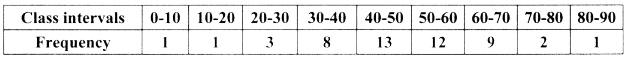We will draw the histogram taking class interval in x-axis and frequency in y-axis as follows.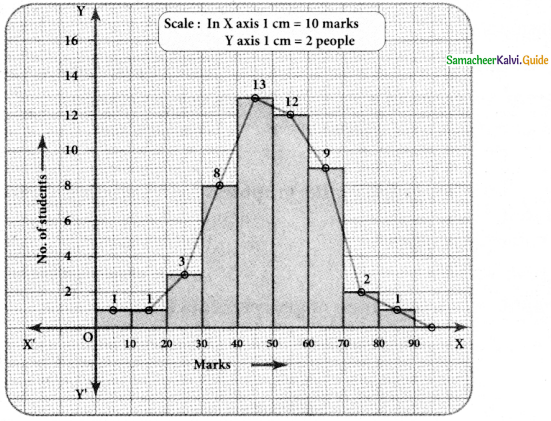Objective Type Questions

Question 9.
Data is a collection of _________
(A) numbers
(B) words
(C) measurements
(D) all the three
(D) all the three

Question 10.
The number of times an observation occurs in the given data is called _________
(A) tally marks
(B) data
(C) frequency
(D) none of these
(C) frequencyQuestion 11.
The difference between the largest value and the smallest value of the given data is ________
(A) range
(B) frequency
(C) variable
(D) none of these
(A) range

Question 12.
The data that can take values between a certain range is called_________
(A) ungrouped
(B) grouped
(C) frequency
(D) none of these
(B) groupedQuestion 13.
Inclusive series is a _________series.
(A) continuous
(B) discontinuous
(C) both
(D) none of these
(B) discontinuous

Question 14.
In a class interval the upper limit of one class is the lower limit of the other class. This is _________ series.
(A) Inclusive
(B) exclusive
(C) ungrouped
(D) none of these
(B) exclusiveQuestion 15.
The graphical representation of ungrouped data is ________
(A) histogram
(B) frequency polygon
(C) pie chart
(D) all the three
(C) pie chart

Question 16.
Histogram is a graph of a ________ frequency distribution.
(A) continuous
(B) discontinuous
(C) discrete
(D) none of these
(A) continuousQuestion 17.
A _________ is a line graph for the graphical representation of the continuous frequency
distribution.
(A) frequency polygon
(B) histogram
(C) pie chart
(D) bar graph